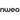# Multiplication charts: 1-12 & 1-100 [Free and printable!]

All Posts

## Printable multiplication chart

We have two multiplication charts available for your class — one for reference and one blank template for students to complete themselves.Get adaptive multiplication practice for your students with Prodigy, a no-cost resource that makes math class engaging.

### Students are constantly learning new and complex content as they progress through their education.

At a young age, they’re introduced to multiplication — something that’s used in everyday life, from simple math when grocery shopping to complex calculations on tax forms.

A simple way to teach students how to multiply is through a multiplication chart or multiplication tables. These charts help students memorize various multiplication equations, so they can come up with answers quickly and accurately.

This article gives you access to free printable multiplication charts for your classroom. We’ll also explain the best ways to teach multiplication tables to your students and show you different multiplication games to help them memorize these tables.

## What is multiplication?

After mastering counting and addition,  students take the big leap to multiplication.

The best way to teach multiplication is to say ‘groups of’ instead of times. Explain to students that when multiplying, they’re adding together groups of numbers.

3 × 4 becomes 3 groups of 4.

Or

4 + 4 + 4 = 12

Multiplication is a shortcut to adding groups of numbers together. This method of thinking helps students understand why they’re multiplying and how it works.

## How to memorize the 1-12 multiplication table

You can teach students several tricks to help them memorize their multiplication charts.

We’ll go through a 1-12 multiplication chart and show you the best tips to teach your students!

### Tips for the 1 times table

Anything multiplied by one stays that number. These equations always mean there’s only one group of numbers.

### Tips for the 2 times table

Anything multiplied by two is being doubled. Students can also think of this as adding two of the same number together.

`6 × 2 is the same as 6 + 6.`

Three doesn’t have any rules that make its multiplication table easy to memorize, but there is a pattern for every ten multiples of three:

`3, 6, 9, 12, 15, 18, 21, 24, 27, 30`

The last digit of these multiples always repeat, which means that students can remember these digits to help them with the three multiplication tables.

Take a look at the next ten multiples of three:

`33, 36, 39, 42, 45, 48, 51, 54, 57, 60`

The last digits in both groups are the same: 3, 6, 9, 2, 5, 8, 1, 4, 7, 0.

If students can remember this order they will at least know what the last digit of any multiplication of three is. For example, numbers that end with a nine that are then multiplied by three are going to then become a number that ends with a seven.

`9 × 3 = 27 19 × 3 = 57 159 × 3 = 477 1,428,659 × 3 = 4,285,977`

Teach your students the pattern of the three multiplication table as if it were a phone number, (369) 258-1470.

### Tips for the 4 times table

When a number is multiplied by four, double it and then double it again. It’s not the most clever trick, but it works!

`8 × 4 becomes 8 + 8 = 16 → 16 +16 = 32.`

### Tips for the 5 times table

Five is one of the easiest multiplication tables to master. Teach students that the five times table always follows the pattern of ending with:

`5, 0, 5, 0, 5, 0...`

Odd numbers multiplied by five are going to end with a five, while even numbers multiplied by five will end with a zero.

### Tips for the 6 times table

When multiplying an even number by six, the solution always ends with the last digit of the number that’s being multiplied.

`2 × 6 = 12        74 × 6 = 444        216 × 6 = 1,296        1,238 × 6 = 7,428`

Unfortunately, this trick only works for even numbers, and not for odd numbers.

We can use our previous tips to figure out the first few multiples of seven:

• Multiplying seven by two is the same as doubling seven — 14
• Three times seven results in a number that ends in a one — 21
• Multiplying seven by four is like doubling it twice — 28
• Multiplying an odd number by five will give a solution that ends in five — 35

But what about remembering later multiples of seven?

Here is a handy trick for seven times eight. The solution for seven times eight is like counting upwards:

`5-6-7-8`
`7 × 8 = 56`

Now your students have another memory trick to help them through their seven times tables.

### Tips for the 8 times table

Double, double, and then double again!

`8 × 8 = 64`
`8 + 8 = 16 → 16 + 16 = 32 → 32 + 32 = 64`

### Tips for the 9 times table

The nine multiplication table seems hard to learn, but there are two tips that can make them simple.

Multiplying by nine is the same as multiplying by ten and then subtracting the other number:

`9 × 5 = 45`

Or

`(10 × 5) - 5 = 50 - 5 = 45`

The second tip works for the first ten multiples of nine. Every time students increase what nine is multiplied with, the tens column of the solution increases by one, while the ones column decreases by one.### Tips for the 10 times table

Ten has the easiest multiplication table to remember. Tell students to add a zero to the end of whichever number they’re multiplying by ten.

```10 × 4 = 40
10 × 7 = 70
10 × 11 = 110
10 × 123 = 1,230```

### Tips for the 11 times table

Up to eleven times nine, tell students they can repeat the digit they’re multiplying by eleven.### Tips for the 12 times table

To make the twelve multiplication table easier, split it into two parts, ten and two. Then add them together!

```12 × 6 = ?
(10 × 6) + (2 × 6) = ?
60 + 12 = 72```

## 4 fun multiplication chart games for the classroom

Students have to remember a lot of numbers when looking at a multiplication chart. Math puzzles and games can make this learning process fun and engaging, which helps ingrain the information in students’ minds.

### 1. Prodigy

Take your students on a virtual adventure, where they can learn important math concepts without realizing they’re practicing math concepts.

Prodigy can help you teach multiplication charts, assign specific questions and track how students are doing in real time — at no cost!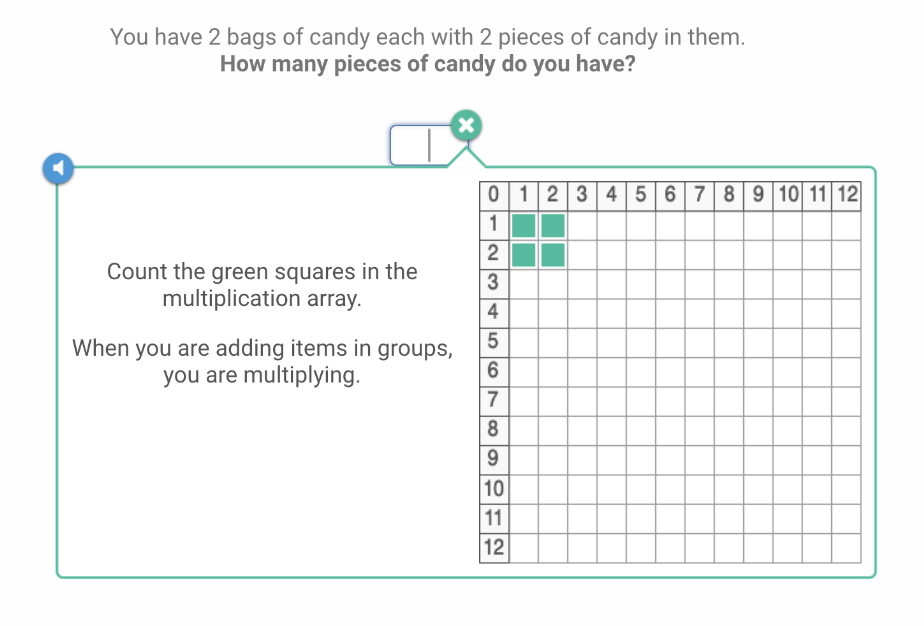The game keeps students engaged with content through an exciting story and loads of features. All of the “marking” is done for you — instantly! Reports can then tell you which topics students are struggling with.

Prodigy creates a personalized learning experience for every student, so they can practice the skills they have trouble with. This allows all students to learn at their own pace.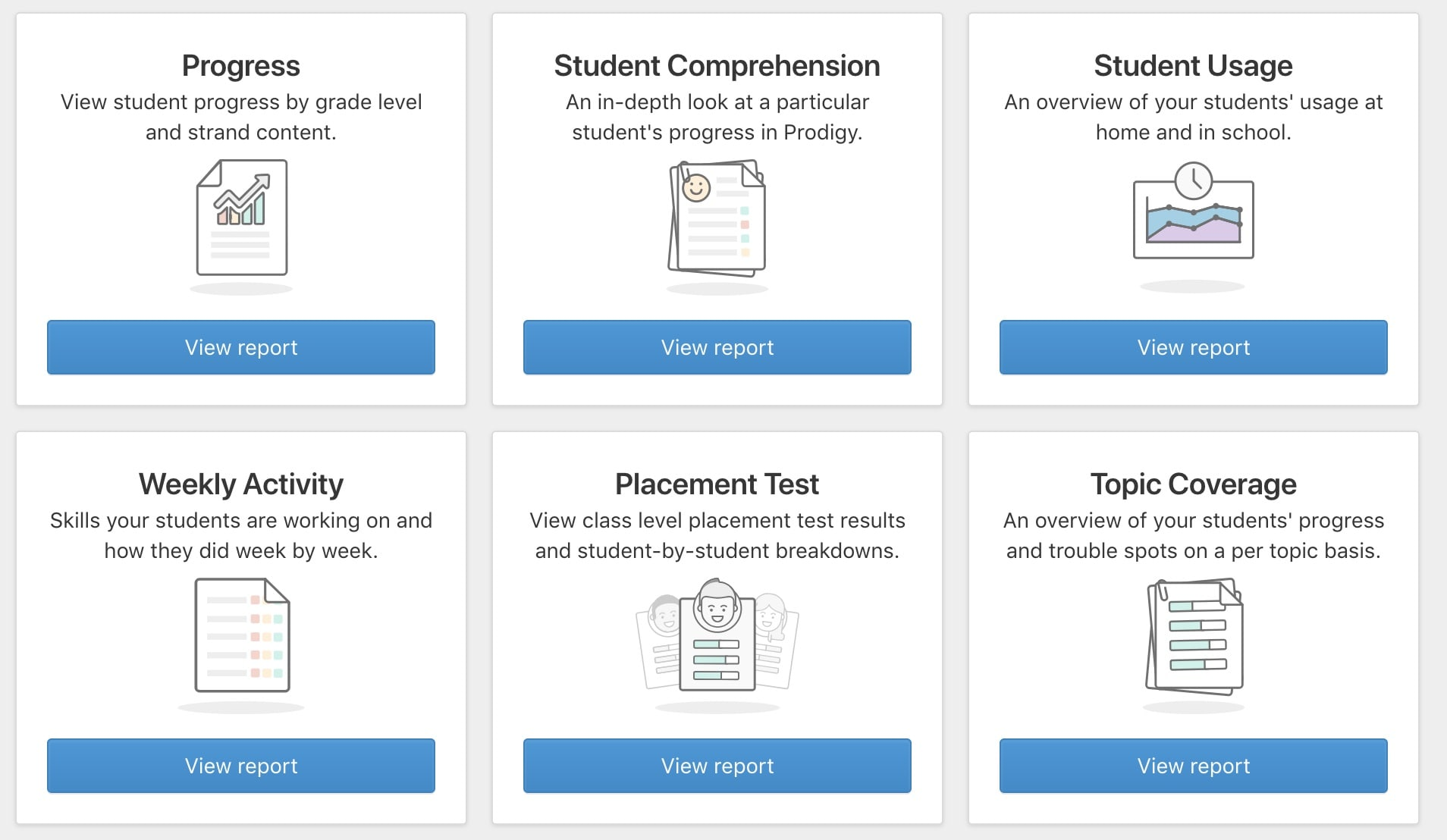Meanwhile, from the teacher dashboard, you can create assignments and practice tests, view statistics, and plan lessons.

Prodigy is a free tool for educators and will remain free — forever!### 2. Table races

In this game, students race against the clock to complete as many equations as possible.

Supply students with a worksheet that has several columns of equations. Each column should have a different multiplication table that’s completely shuffled up. For example, one column will only have the four multiplication table while the next has the seven multiplication table.

Keep the equations shuffled so students can’t simply count upwards to get the answer. They’ll need to think critically as answers range across the different multiplication tables they’ve been taught.Once every student has a worksheet, give them a countdown of 60 seconds to answer 20 questions. Then have students tally up their answers to see how they did.

This exercise is just for fun, but adding an incentive — like crowning a class champion — can get students more interested in the activity. Try to do this activity a couple of times a week.

Eventually, students will have no trouble filling out entire columns of questions correctly in less than 60 seconds!

Try this free table race template in your class!

### 3. Multiplication bingo

This game can be played as a class or in groups. You’ll either need dice or a random number generator, and bingo sheets to get started. Bingo sheets will have the solutions to multiplication chart equations in a five-by-five grid.

You can fill out these charts yourself, or have students create their own bingo sheets to get them a little more involved.

Note: if you’re using dice, make sure your students aren’t putting the one multiplication tables in their bingo sheet, as it will be impossible for those to come up.

Save Free, printable Multiplication Bingo Cards (30)Prodigy Education Math Lessons & Math Resources

Now, use dice or a random number generator to get your equations. Students then solve the equations to get the numbers on their bingo cards.

If students are working in groups, have them write down the equations so you can check their work.

For dice, roll a pair of them and add the values to get the first number. Then roll the pair of dice again and add the values together to get the number to multiply by. For the random number generator, randomize two numbers from 1-12 and use them for the equation to solve.

Once students have solved the equation they can check their bingo sheet to see if the value is there. This is a great way to get students to think critically and avoid simple memorization of multiplication tables in list form.

### 4. Multiply to the top

This game creates a class competition to see who can complete their multiplication tables the quickest — and more importantly, the most accurate.

Shuffle up the entire 1-12 multiplication chart and have students compete to see who can answer questions the fastest. The trick is, as soon as a student answers a question wrong, their climb is over. This ensures students aren’t just trying to answer as fast as possible, but are making sure their answers are correct.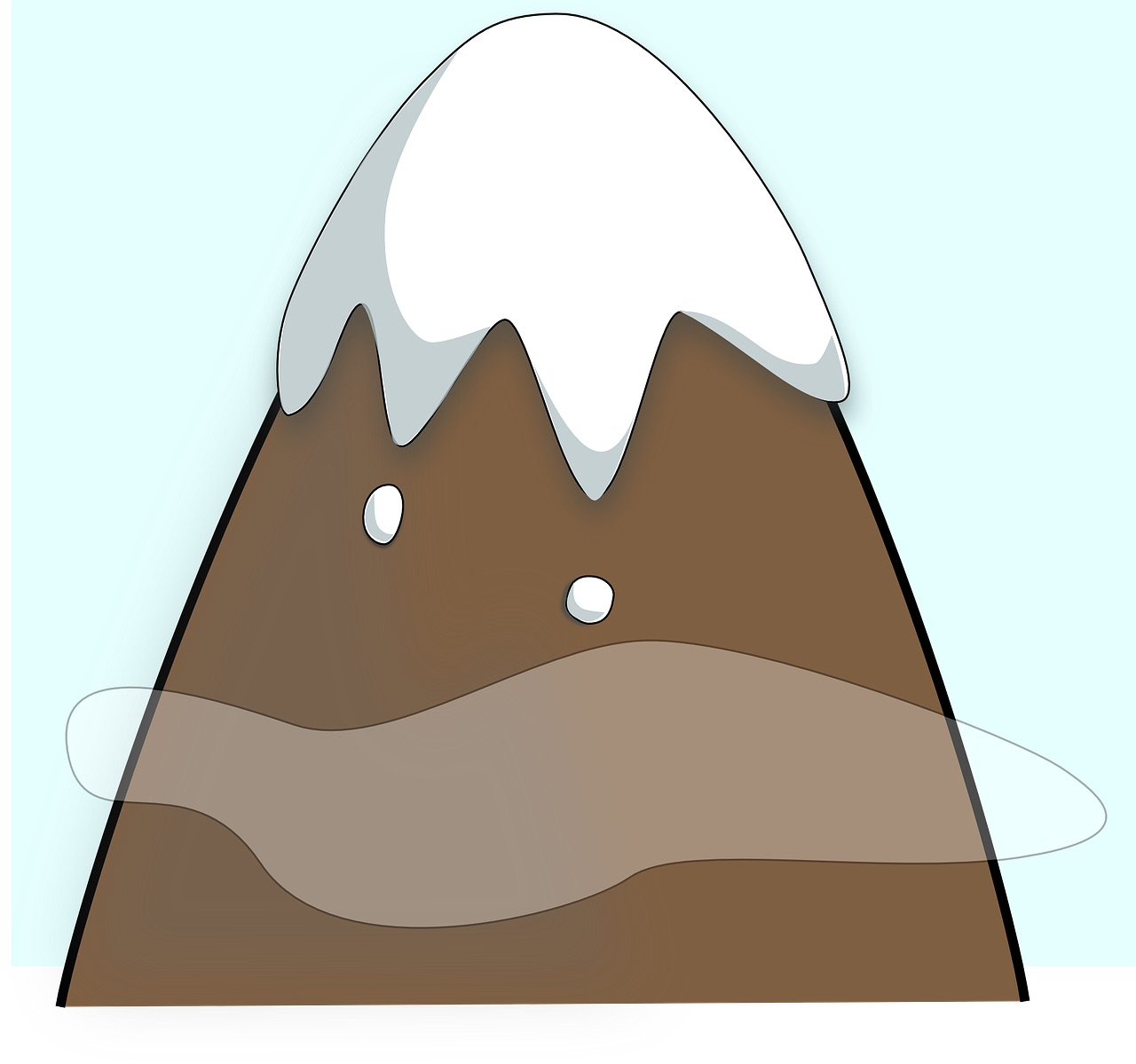Create a visual board for students to look at with a picture of a mountain. Put students’ names up the mountain, depending on how far they got. Students will love competing to reach the top.

Make an event of this game. Students can answer questions at the front of the class to try for the mountaintop title. Students will be excited to compete and showcase their skills.

## Conclusion: multiplication table chart

Multiplication is one of the most common forms of math that we encounter every day.

Learning the basic multiplication chart is essential for young students. It will help them throughout their educational career and their daily lives.

Use our free printable multiplication chart 1-12 in your classroom to teach your students how to do simple multiplication quickly and flawlessly. Our list of tips and games makes multiplication easy and fun.

•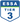•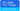•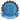•